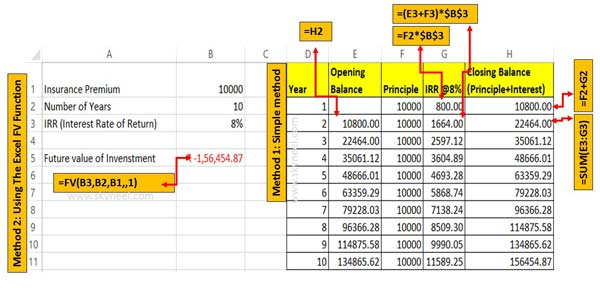# Excel FV Function to know future value of an Investment

Excel FV Function in an important financial function which allows you to know future value of an Investment based on constant payment, rate.

Hi friends today we are talking on Excel FV Function to know future value of an Investment. The Excel FV function is an important financial function. The Excel FV function allows you to quickly return the future value of an investment with constant interest rate, payments and periodic. FV stand for future values and this function is located in Formula > Financial library functions.

For Example:

In the given spreadsheet, the Excel FV function is used to calculate the future value of an investment against the insurance premium. The principle amount of insurance premium is \$10000 per year for the period of 10 years. The annual interest rate is 8% for this investment. Now you want to know how much amount you will get after complete the 10 years against the investment.

Before we start how to use The Excel FV Function to know future value of an Investment, you must have to know few things.

## The Excel FV Function to know future value of an Investment

To get the future value of an investment you must have to use Excel FV function in active worksheet.

Syntax of Excel FV function: FV(rate,nper,pmt,[pv],[type])

Important Arguments of FV function:

Rate: The required interest rate per period.

Nper: The required total number of payment periods in an annuity.

Pmt: The payment made each period; it cannot change over the life of the annuity. It is an required argument.

Pv: It’s an option argument.The present value, or the lump-sum amount that a series of future payments is worth right now.

Type: It is another an optional argument. The number 0 or 1 and indicates when payments are due. If type is omitted, it is assumed to be 0. 0 means at the end of the period while 1 at the beginning of the period.### Method 1: Simple method to know future value of an Investment

This is the simple method which allows you to know future value of an Investment without using the Excel FV function. You have to take given simple steps to get the result, have a look.

Step 1: Create the following structure to get the future value of investment.

Step 2: Calculate first year interest amount using given function in cell G2 =F2*\$B\$3. Just like this calculate closing balance (Principle+Interest) in cell H2 =F2+G2 and press enter. The closing balance will be the opening balance for next year instalment.

Step 3: Now, you can calculate second year interest amount as per the given formula in cell G3 =(E3+F3)*\$B\$3. Like this you can calculate the closing balance in H3 using the given formula =SUM(E3:G3). Now drag or copy the formula of cell G3 and H3 to rest of the cells. Finally you can check at the end of last year insurance premium instalment you will get the final return of an investment.

### Method 2: Using The Excel FV – Future Value Function to calculate return of investment

This is another way in which you can use FV function to calculate the return of investment. To get the future value against the investment for specific period, interest rate you have to take following given steps.

Step 1: Create the following structure to get the future value of investment.

Step 2: Apply the following given function in cell B5 =FV(B3,B2,B1,,1). Now press enter to get the future value of an investment. You can check both methods shown same result against the return of an investment.

You can choose any method to get the result against your investment. If you have any suggestion regarding this article then please write us in the comment box. Thanks to all.